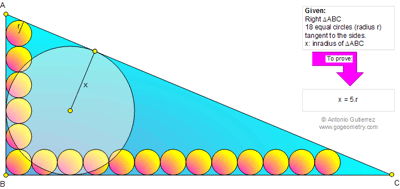## Thursday, July 19, 2012

### Problem 787: Right Triangle, Inradius, 18 Equal Circles, Line Tangent, Legs, Hypotenuse, Ratio, Metric Relations

Geometry Problem
Level: Mathematics Education, High School, Honors Geometry, College.

Click the figure below to see the complete problem 787.1.http://img836.imageshack.us/img836/5494/problem787.png

Draw lines per attached sketch
We have A’B’=10r
B’C’=24r
And A’C’=26r …. ( Pythagoras theorem)
Half of perimeter of A’B’C’= p= (10r+4r+26r)/2=30r
And radius of internal circle= p-A’C’= 4r
So x= x’+r=5r

2.Let AB=a, BC=b.

From two pairs of similar triangles, we have
(1): x/(a-x) = r/(a-11r)
(2): x/(b-x) = r/(b-25r)

(1) simplifies to: a(x-r) = 10rx ... (3)
(2) simplifies to b(x-r) = 24rx ... (4)

(3)/(4): a/b = 5/12

WLOG, let a=5, b=12. Then x=2.

Substitude into (3) or (4),
from (3): 5(2-r) = 20r
from (4): 12(2-r) = 48r

Both of them are equivalent to
2-r = 4r
r = 2/5

Hence, x=5r.

3.From the figure: a=25r+r*cot(C/2) ---(1) & c=11r+r*cot(A/2)---(2). It's reasonably easy to show that:cot(A/2)=c/(b-a) while cot(A/2)=a/(b-c) which we can substitute in (1)&(2) to obtain:[25(b-a)+c]/[11(b-c)+a)]=[a(b-a)/c(b-c)]. We've b^2=a^2+c^2 as well which yields:a=12b/3 & c=5b/13
For a right triangle: x=(a+c-b)/2 or x=2b/13.
We can also plug in values of a and cot(C/2) in equation (1) to obtain r=a/30=2b/65 or x=5r

4.Draw tangents to the last little circles,// to BC at A;// to AB at C,we obtain 2 congruent triangles similar to ABC,so:
c=10r+kc and a=24r+ka and r=kx
hence
a=24t;c=10t (t positive real number)
ABC is a right triangle b=26t
with the area x=4t
this gives k=1/5
x=5r

5.Let A’, B’, C’ be the centres of the smaller circles (each of radius r) nearest to A, B, C respectively.
Consider triangle A’B’C’. It is right angled at B’
A’B’ = 10r, B’C’ = 24r. Follows A’C’ = 26r by Pythagoras.
AA’, BB’, CC’ concur at the (common) incentre I of the similar and similarly situated right triangles ABC and A’B’C’.
Let x’ be the inradius of the right triangle A’B’C’.
Clearly 2x’ = A’B’ + B’C’ – A’C’ = 8r and
Hence x = x’ + r = 4r + r = 5r